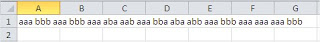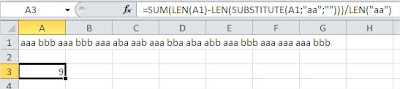#### How to Count Repeated Words in Excel

In this Excel tutorial lesson, you will learn some trick – how to count repeated words. I implemented a word counter and would like to share it with you.

## Counting words in cell

There is a task. How to count repeated words in a cell?

For example, you have some words (e.g. aa) which repeat many times in one cell.Use that function:

=SUM(LEN(A1)-LEN(SUBSTITUTE(A1;”aa”;””)))/LEN(“aa”)The Word Counter worked. There are 9 “aa” words.

## Counting words in column

To count repeated words in a column, you can use the combination of the IF and COUNTIF functions. This method identifies and lists the repeated words in a separate column by comparing each cell with all others in the same column. Here’s a summary of the steps:

In a separate column, write a formula to compare each cell in the column containing your words with all other cells in that same column:

=IF(COUNTIF(A:A, A1)>1, A1, “”)

Drag the formula down to the last row of your data.

Filter the new column for non-empty cells, which will show only the repeated words.

This method is useful when you have a list of words in a column, and you want to identify and count the words that appear more than once.

Note that this method assumes that the words are in separate cells in the same column. If they are in a single cell, you’ll need to use a different approach, such as using a macro or splitting the cell into separate cells before using the above method.